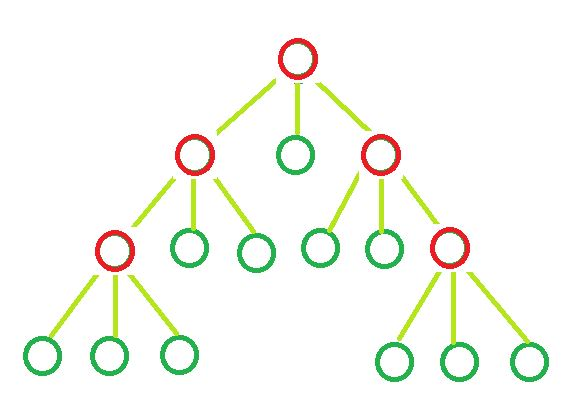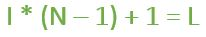# Determine the count of Leaf nodes in an N-ary tree

Given the value of ‘N’ and ‘I’. Here,represents the number of internal nodes present in an N-ary tree and every node of the N-ary can either havechilds or zero child. The task is to determine the number of Leaf nodes in n-ary tree.

Examples:

Input : N = 3, I = 5
Output : Leaf nodes = 11Input : N = 10, I = 10
Output : leaf nodes = 91

## Recommended: Please try your approach on {IDE} first, before moving on to the solution.

Formula:where,
I = Number of Internal nodes.
L = Leaf Nodes.
and, N = Number of children each node can have.

Derivation: The tree is an N-ary tree. Assume it has T total nodes, which is the sum of internal nodes (I) and leaf nodes (L). A tree with T total nodes will have (T – 1) edges or branches.

In other words, since the tree is an N-ary tree, each internal node will have N branches contributing a total of N*I internal branches. Therefore we have the following relations from the above explanations,

• N * I = T – 1
• L + I = T

From the above two equations, we can say that L = (N – 1) * I + 1.

Below is the implementation of the above approach:

## C++

 `// CPP program to find number ` `// of leaf nodes ` ` `  `#include ` `using` `namespace` `std; ` ` `  `// Function to calculate ` `// leaf nodes in n-ary tree ` `int` `calcNodes(``int` `N, ``int` `I) ` `{ ` `    ``int` `result = 0; ` ` `  `    ``result = I * (N - 1) + 1; ` ` `  `    ``return` `result; ` `} ` ` `  `// Driver code ` `int` `main() ` `{ ` `    ``int` `N = 5, I = 2; ` ` `  `    ``cout << ``"Leaf nodes = "` `<< calcNodes(N, I); ` ` `  `    ``return` `0; ` `} `

## Java

 `// Java program to find number  ` `// of leaf nodes  ` ` `  `class` `GfG  ` `{ ` ` `  `// Function to calculate  ` `// leaf nodes in n-ary tree  ` `static` `int` `calcNodes(``int` `N, ``int` `I)  ` `{  ` `    ``int` `result = ``0``;  ` ` `  `    ``result = I * (N - ``1``) + ``1``;  ` ` `  `    ``return` `result;  ` `}  ` ` `  `// Driver code  ` `public` `static` `void` `main(String[] args)  ` `{  ` `    ``int` `N = ``5``, I = ``2``;  ` ` `  `    ``System.out.println(``"Leaf nodes = "` `+  ` `                        ``calcNodes(N, I));  ` `} ` `}  ` ` `  `// This code is contributed by Prerna Saini `

## Python3

 `# Python3 program to find number  ` `# of leaf nodes  ` ` `  `# Function to calculate  ` `# leaf nodes in n-ary tree  ` `def` `calcNodes(N, I): ` `    ``result ``=` `0` ` `  `    ``result ``=` `I ``*` `(N ``-` `1``) ``+` `1` ` `  `    ``return` `result  ` ` `  `# Driver Code  ` `if` `__name__ ``=``=` `'__main__'``: ` `    ``N ``=` `5` `    ``I ``=` `2` ` `  `    ``print``(``"Leaf nodes = "``,  ` `           ``calcNodes(N, I)) ` ` `  `# This code is contributed  ` `# by SHUBHAMSINGH10 `

## C#

 `// C# program to find number  ` `// of leaf nodes  ` `using` `System; ` ` `  `class` `GFG  ` `{ ` ` `  `// Function to calculate  ` `// leaf nodes in n-ary tree  ` `static` `int` `calcNodes(``int` `N, ``int` `I)  ` `{  ` `    ``int` `result = 0;  ` ` `  `    ``result = I * (N - 1) + 1;  ` ` `  `    ``return` `result;  ` `}  ` ` `  `// Driver code  ` `public` `static` `void` `Main()  ` `{  ` `    ``int` `N = 5, I = 2;  ` ` `  `    ``Console.Write(``"Leaf nodes = "` `+  ` `                  ``calcNodes(N, I));  ` `} ` `}  ` ` `  `// This code is contributed ` `// by Akanksha Rai `

## PHP

 ` `

Output:

```Leaf nodes = 9
```

Don’t stop now and take your learning to the next level. Learn all the important concepts of Data Structures and Algorithms with the help of the most trusted course: DSA Self Paced. Become industry ready at a student-friendly price.

My Personal Notes arrow_drop_upCheck out this Author's contributed articles.

If you like GeeksforGeeks and would like to contribute, you can also write an article using contribute.geeksforgeeks.org or mail your article to contribute@geeksforgeeks.org. See your article appearing on the GeeksforGeeks main page and help other Geeks.

Please Improve this article if you find anything incorrect by clicking on the "Improve Article" button below.